# pcregistericp

Register two point clouds using ICP algorithm

## Syntax

``tform = pcregistericp(moving,fixed)``
``````[tform,movingReg] = pcregistericp(moving,fixed)``````
``[___,rmse] = pcregistericp(moving,fixed)``
``[___] = pcregistericp(moving,fixed,Name=Value)``

## Description

example

````tform = pcregistericp(moving,fixed)` returns a rigid transformation that registers a moving point cloud to a fixed point cloud.The registration algorithm is based on the "iterative closest point" (ICP) algorithm. Best performance of this iterative process requires adjusting properties for your data. To improve the accuracy and efficiency of registration, consider downsampling point clouds using `pcdownsample` before using `pcregistericp`.The registration algorithm requires point cloud normals when you select the `"pointToPlane"` or the `"planeToPlane"` (also known as Generalized-ICP or G-ICP) metric. If the `Normal` property of the input point cloud is empty, the function fills it.```
``````[tform,movingReg] = pcregistericp(moving,fixed)``` additionally returns the transformed point cloud that aligns with the fixed point cloud.```
````[___,rmse] = pcregistericp(moving,fixed)` additionally returns the root mean square error of the Euclidean distance between the inlier aligned points, using any of the preceding syntaxes.```
````[___] = pcregistericp(moving,fixed,Name=Value)` specifies options using one or more name-value arguments in addition to any combination of arguments from previous syntaxes. For example, `pcregistericp(moving,fixed,Metric="planeToPlane")` sets the minimization metric to `"planeToPlane"`.```

## Examples

collapse all

Load point cloud data into the workspace.

`ptCloud = pcread("teapot.ply");`

Display the loaded point cloud.

```pcshow(ptCloud) title("Teapot")```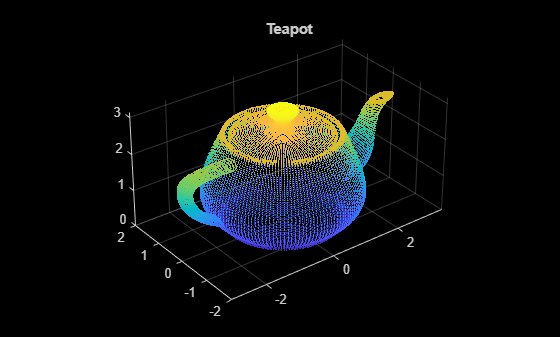Create a transformation object with 30 degree rotation along the Z-axis and with a translation of [5 5 10].

```rotationAngles = [0 0 30]; translation = [5 5 10]; tform1 = rigidtform3d(rotationAngles,translation);```

Transform the point cloud and visualize the result.

```ptCloudTformed = pctransform(ptCloud,tform1); pcshow(ptCloudTformed) title("Transformed Teapot")```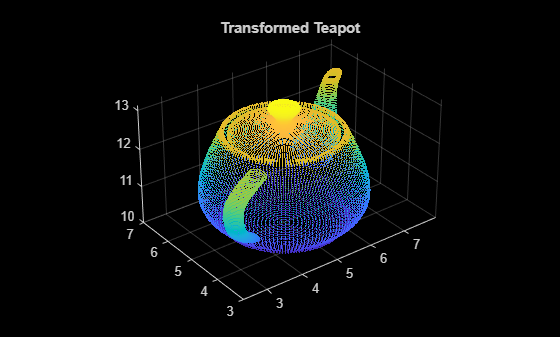Register the point clouds.

`tform = pcregistericp(ptCloudTformed,ptCloud,Extrapolate=true);`

Compare the result with the true transformation.

`disp(tform1.A);`
``` 0.8660 -0.5000 0 5.0000 0.5000 0.8660 0 5.0000 0 0 1.0000 10.0000 0 0 0 1.0000 ```
```tform2 = invert(tform); disp(tform2.A);```
``` 0.8660 -0.5000 -0.0000 5.0000 0.5000 0.8660 -0.0000 5.0000 0.0000 0.0000 1.0000 10.0000 0 0 0 1.0000 ```

Load point cloud data into the workspace.

```ld = load("livingRoom.mat"); moving = ld.livingRoomData{1}; fixed = ld.livingRoomData{2};```

Visualize the point cloud and set the direction to display the y-axis.

```figure pcshowpair(moving,fixed,VerticalAxis="Y",VerticalAxisDir="Down")```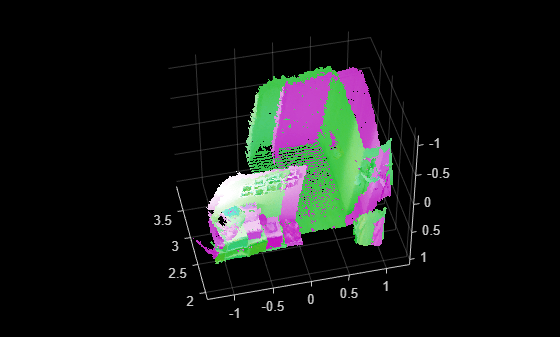Downsample the point clouds using `nonuniformGridSample` method to improve the efficiency and accuracy of registration.

```maxNumPoints = 12; fixedDownsampled = pcdownsample(fixed,"nonuniformGridSample",maxNumPoints); movingDownsampled = pcdownsample(moving,"nonuniformGridSample",maxNumPoints);```

Align the point clouds using plane-to-plane (Generalized-ICP) registration.

`[~,movingReg] = pcregistericp(movingDownsampled,fixedDownsampled,Metric="planeToPlane");`

Visualize the point cloud alignment and set the direction to display the y-axis.

```figure pcshowpair(movingReg,fixed,VerticalAxis="Y",VerticalAxisDir="Down")```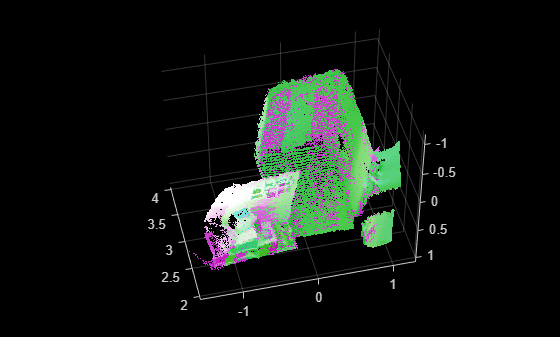## Input Arguments

collapse all

Moving point cloud, specified as a `pointCloud` object.

Fixed point cloud, specified as a `pointCloud` object.

### Name-Value Arguments

Specify optional pairs of arguments as `Name1=Value1,...,NameN=ValueN`, where `Name` is the argument name and `Value` is the corresponding value. Name-value arguments must appear after other arguments, but the order of the pairs does not matter.

Before R2021a, use commas to separate each name and value, and enclose `Name` in quotes.

Example: `"planeToPlane"` sets the minimization metric for the ICP algorithm to `"planeToPlane"`.

Minimization metric, specified as `"pointToPoint"`, `"pointToPlane"`, or `"planeToPlane"`. The iterative closest point (ICP) algorithm estimates the rigid transformation between the moving and fixed point clouds by minimizing the distance between the two point clouds according to the specified metric.

Specifying `Metric` as `"pointToPlane"` or `"planeToPlane"` (also known as the Generalized-ICP algorithm) can reduce the number of iterations required for registration. However, these metrics require extra algorithmic steps within each iteration. Use the `"pointToPlane"` or the `"planeToPlane"` metric to improve the registration of planar surfaces.

Downsample Method Selection
Downsample the point clouds using the `pcdownsample` function. The registration algorithm requires point cloud normals when you select the `"pointToPoint"` metric. The `"nonuniformGridSample"` algorithm computes the normals on the original data prior to downsampling, resulting in more accurate normals. Therefore, using the `"nonuniformGridSample"` downsampling method can result in a more accurate `"pointToPoint"` registration. Use the `"random"`, `"gridAverage"`, or `"nonuniformGridSample"` method for the `pcdownsample` function according to this table.

MetricMoving Point Cloud Downsample MethodFixed Point Cloud Downsample Method
`"pointToPoint"``"random"``"random"`
`"gridAverage"``"gridAverage"`
`"pointToPlane"``"gridAverage"``"gridAverage"`
`"random"``"nonuniformGridSample"`
`"planeToPlane"``"gridAverage"``"gridAverage"`
`"nonuniformGridSample"``"nonuniformGridSample"`

Extrapolation, specified as a logical `0` (`false`) or `1` (`true`). When you specify this argument as `true`, the function adds an extrapolation step that traces out a path in the registration state space, as described in A Method for Registration of 3-D Shapes. Enabling extrapolation can reduce the number of iterations required for the point clouds to converge. You cannot specify the `Extrapolate` name-value argument when using the `"planeToPlane"` metric.

Percentage of inliers, specified as a scalar between (0, 1]. This value specifies the percentage of matched pairs to use as inliers. The function considers a pair of matched points to be an inlier if the Euclidean distance between the two points is less than the specified percent of the maximum Euclidean distance between any two points in a matched pair of the point clouds. By default, the function uses all matching pairs.

Maximum number of iterations, specified as a positive integer. This value specifies the maximum number of iterations over which the function attempts to make the two point clouds converge.

For the `"pointToPoint"` and `"pointToPlane"` metrics, the default value of this argument is `20`. For the `"planeToPlane"` metric, the default value is 30.

Tolerance between consecutive ICP iterations, specified as a two-element vector of the form [Tdiff Rdiff], where Tdiff and Rdiff represent the tolerances of absolute difference in translation and in rotation, respectively, estimated in consecutive ICP iterations. Tdiff measures the Euclidean distance between two translation vectors. Rdiff measures the angular difference in degrees. The algorithm stops when the average difference between estimated rigid transformations in the three most recent consecutive iterations is less than the specified tolerance values.

Initial rigid transformation, specified as a `rigidtform3d` object. The initial rigid transformation is useful when you provide an external coarse estimation.

Display progress information, specified as a logical `0` (`false`) or `1` (`true`). Specify `Verbose` as `true` to display progress information.

## Output Arguments

collapse all

Rigid transformation, returned as a `rigidtform3d` object. The rigid transformation registers a moving point cloud to a fixed point cloud. The `rigidtform3d` object describes the rigid 3-D transform. The iterative closest point (ICP) algorithm estimates the rigid transformation between the moving and fixed point clouds.

Transformed point cloud, returned as a `pointCloud` object. The transformed point cloud is aligned with the fixed point cloud.

Root mean square error, returned as a positive numeric scalar that represents the Euclidean distance between the inlier aligned points.

## Algorithms

collapse all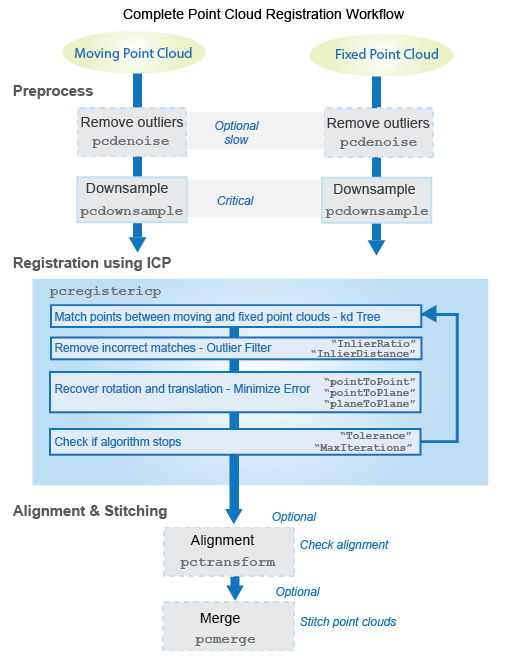### Tips

 Besl, P.J., and Neil D. McKay. “A Method for Registration of 3-D Shapes.” IEEE Transactions on Pattern Analysis and Machine Intelligence 14, no. 2 (February 1992): 239–256. https://doi.org/10.1109/34.121791.

 Chen, Yang, and Gérard Medioni. “Object Modelling by Registration of Multiple Range Images.” Image and Vision Computing 10, no. 3 (April 1992): 145–155. https://doi.org/10.1016/0262-8856(92)90066-C.

 Segal, A., Haehnel, D. and S. Thrun. "Generalized-ICP". Robotics: Science and Systems V, Robotics: Science and Systems Foundation,. (June 2009): 435-442. https://doi.org/10.15607/RSS.2009.V.021.